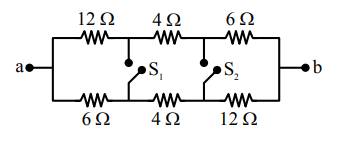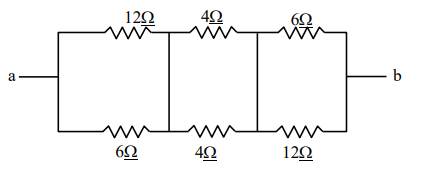# In the given figure switches`
Question:

In the given figure switches $S_{1}$ and $S_{2}$ are in open condition. The resistance across ab when the Switches $S_{1}$ and $S_{2}$ are closed is________ $\Omega .$Solution:

when switch $S_{1}$ and $S_{2}$ are closed$\frac{12 \times 6}{12+6}+2+\frac{6 \times 12}{6+12}$

$\frac{72}{18}+2+\frac{72}{18}=4+2+4=10 \Omega$